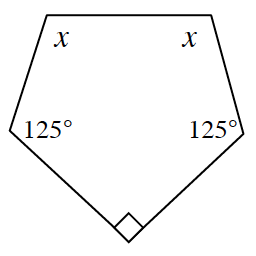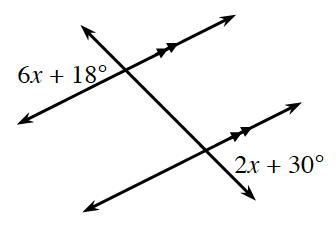### Home > INT2 > Chapter 8 > Lesson 8.3.2 > Problem8-73

8-73.

For each diagram below, write and solve an equation for $x$.

1.The sum of the interior angles $= 180^\circ(n - 2)$.

$x + x + 125^\circ + 90^\circ + 125^\circ =$ sum from Step $1$

1.Mark the angles that are vertical to the labeled angles. What do you know about the angles that you marked?

$x = 3^\circ$